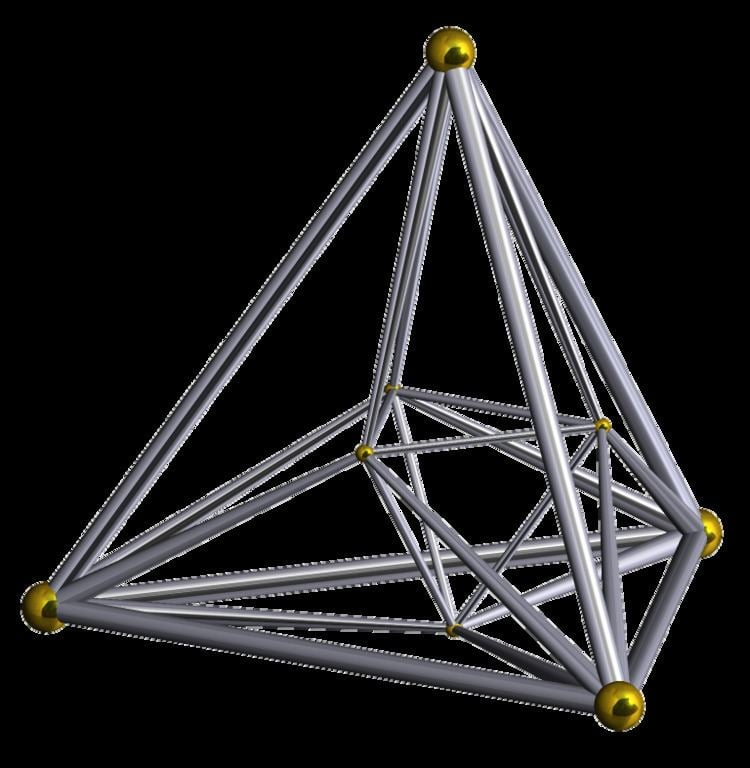# 16 cell

Updated on
Covid-19
 Schläfli symbol  {3,3,4}Faces  32 {3}Vertices  8 Cells  16 {3,3}Edges  24Type  Convex regular 4-polytope 4-orthoplex 4-demicube

In four-dimensional geometry, a 16-cell is a regular convex 4-polytope. It is one of the six regular convex 4-polytopes first described by the Swiss mathematician Ludwig Schläfli in the mid-19th century. It is also called C16, hexadecachoron, or hexdecahedroid.

## Contents

It is a part of an infinite family of polytopes, called cross-polytopes or orthoplexes. The dual polytope is the tesseract (4-cube). Conway's name for a cross-polytope is orthoplex, for orthant complex. The 16-cell has 16 cells as the tesseract has 16 vertices.

## Geometry

It is bounded by 16 cells, all of which are regular tetrahedra. It has 32 triangular faces, 24 edges, and 8 vertices. The 24 edges bound 6 squares lying in the 6 coordinate planes.

The eight vertices of the 16-cell are (±1, 0, 0, 0), (0, ±1, 0, 0), (0, 0, ±1, 0), (0, 0, 0, ±1). All vertices are connected by edges except opposite pairs.

The Schläfli symbol of the 16-cell is {3,3,4}. Its vertex figure is a regular octahedron. There are 8 tetrahedra, 12 triangles, and 6 edges meeting at every vertex. Its edge figure is a square. There are 4 tetrahedra and 4 triangles meeting at every edge.

The 16-cell can be decomposed into two similar disjoint circular chains of eight tetrahedrons each, four edges long. Each chain, when stretched out straight, forms a Boerdijk–Coxeter helix. This decomposition can be seen in a 4-4 duoantiprism construction of the 16-cell: or , Schläfli symbol {2}⨂{2} or s{2}s{2}, symmetry [[4,2+,4]], order 64.

The 16-cell can be dissected into two octahedral pyramids, which share a new octahedron base through the 16-cell center.

## Tessellations

One can tessellate 4-dimensional Euclidean space by regular 16-cells. This is called the 16-cell honeycomb and has Schläfli symbol {3,3,4,3}. Hence, the 16-cell has a dihedral angle of 120°. The dual tessellation, 24-cell honeycomb, {3,4,3,3}, is made of by regular 24-cells. Together with the tesseractic honeycomb {4,3,3,4}, these are the only three regular tessellations of R4. Each 16-cell has 16 neighbors with which it shares a tetrahedron, 24 neighbors with which it shares only an edge, and 72 neighbors with which it shares only a single point. Twenty-four 16-cells meet at any given vertex in this tessellation.

## Boerdijk–Coxeter helix

A 16-cell can constructed from two Boerdijk–Coxeter helixes of eight chained tetrahedra, each folded into a 4-dimensional ring. The 16 triangle faces can be seen in a 2D net within a triangular tiling, with 6 triangles around every vertex. The purple edges represent the Petrie polygon of the 16-cell.

## Projections

The cell-first parallel projection of the 16-cell into 3-space has a cubical envelope. The closest and farthest cells are projected to inscribed tetrahedra within the cube, corresponding with the two possible ways to inscribe a regular tetrahedron in a cube. Surrounding each of these tetrahedra are 4 other (non-regular) tetrahedral volumes that are the images of the 4 surrounding tetrahedral cells, filling up the space between the inscribed tetrahedron and the cube. The remaining 6 cells are projected onto the square faces of the cube. In this projection of the 16-cell, all its edges lie on the faces of the cubical envelope.

The cell-first perspective projection of the 16-cell into 3-space has a triakis tetrahedral envelope. The layout of the cells within this envelope are analogous to that of the cell-first parallel projection.

The vertex-first parallel projection of the 16-cell into 3-space has an octahedral envelope. This octahedron can be divided into 8 tetrahedral volumes, by cutting along the coordinate planes. Each of these volumes is the image of a pair of cells in the 16-cell. The closest vertex of the 16-cell to the viewer projects onto the center of the octahedron.

Finally the edge-first parallel projection has a shortened octahedral envelope, and the face-first parallel projection has a hexagonal bipyramidal envelope.

## 4 sphere Venn Diagram

The usual projection of the 16-cell and 4 intersecting spheres (a Venn diagram of 4 sets) form topologically the same object in 3D-space:

## Symmetry constructions

There is a lower symmetry form of the 16-cell, called a demitesseract or 4-demicube, a member of the demihypercube family, and represented by h{4,3,3}, and Coxeter diagrams or . It can be drawn bicolored with alternating tetrahedral cells.

It can also be seen in lower symmetry form as a tetrahedral antiprism, constructed by 2 parallel tetrahedra in dual configurations, connected by 8 (possibly elongated) tetrahedra. It is represented by s{2,4,3}, and Coxeter diagram: .

It can also be seen as a snub 4-orthotope, represented by s{21,1,1}, and Coxeter diagram: or .

With the tesseract constructed as a 4-4 duoprism, the 16-cell can be seen as its dual, a 4-4 duopyramid.

## Related complex polygons

The Möbius–Kantor polygon is a regular complex polygon 3{3}3, , in C 2 shares the same vertices as the 16-cell. It has 8 vertices, and 8 3-edges.

The regular complex polygon, 2{4}4, , in C 2 has a real representation as a 16-cell in 4-dimensional space with 8 vertices, 16 2-edges, only half of the edges of the 16-cell. Its symmetry is 42, order 32.

## Related uniform polytopes and honeycombs

The regular 16-cell along with the tesseract exist in a set of 15 uniform 4-polytopes with the same symmetry. It is also a part of the uniform polytopes of D4 symmery.

This 4-polytope is also related to the cubic honeycomb, order-4 dodecahedral honeycomb, and order-4 hexagonal tiling honeycomb which all have octahedral vertex figures.

It is in a sequence to three regular 4-polytopes: the 5-cell {3,3,3}, 600-cell {3,3,5} of Euclidean 4-space, and the order-6 tetrahedral honeycomb {3,3,6} of hyperbolic space. All of these have a tetrahedral cells.

It is first in a sequence of quasiregular polytopes and honeycombs h{4,p,q}, and a half symmetry sequence, for regular forms {p,3,4}.

## References

Topics

 B i Link H2
 L